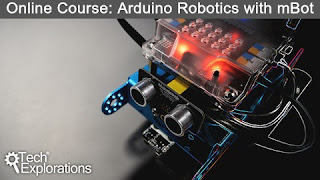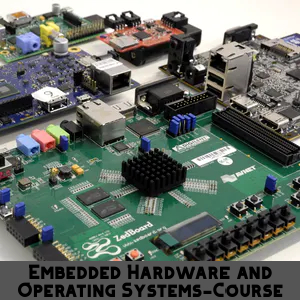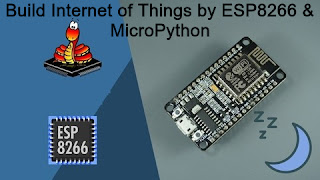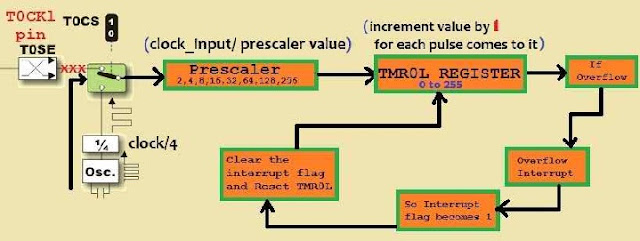# Learn Embedded Programming [Step by Step]

## Embedded Programming Tutorials for Beginners with Step By Step Guide.## Time Calculation:Part-4

In this part we will connect a push button with microcontroller which will create an external interrupt and we will calculate the time duration of that interrupt .

## Calculation :

Now , we need to know how to calculate duration time of an event .IR (Infrared) Remote Controlled Communication Between Two Microcontroller -Step By Step Tutorial : Part-4

First, we  know that clock frequency will be divided by 4 and secondly, it will go to prescalar. Finally, clock goes to timer register.
So, if we use 12MHz clock and 1:4 prescalar. First we will get system clock (12/4)=3MHz clock or (12000000/4)=3000000Hz clock. Secondly it goes to prescalar and divided by 4 again. So we get (3000000/4)=750000Hz clock.
We know that for each pulse TMR0L(Timer0) register is incremented by one. So when 750000 Hz clock successfully go to timer0 register and the TMR0L register increments for 750000 times. Is it clear? We have a problem here, our TMR0L register can only count from 0 to 255.
As we need to count 750000 or greater than 750000. We have to walk in another way. We will set an integer variable count which will be incremented for each overflow interrupt. That means, when TMR0L counts 255 and overflow interrupt occurs. So it gets reset and count is incremented by one. Actually when overflow interrupt occurs, it increases the value of count variable by one. That means count=count+1;

Now consider an External Interrupt situation on RB0 pin where count=3 and TMR0L(8bit Register)=123.

How many pulses has been passed on that External Interrupt situation ?
Total pulses will be [On that situation]
tp = (count * 255) + TMR0L;

Think about that, We are using 12MHz clock and finally we get 750000Hz clock from 12MHz clock.

#### So , What is the meaning of this 750000 Hz clock frequency ?

It means, when 750000 pulses have been received, time is 1 second. We read the definition of frequency in physics. So the Time period t =(1/f);  The time required for one pulse.

### Right Now, we can say that :

tp=(count * 255)+ TMR0L ;

750000 total pulses means = 1 second

so   1    ,,     ,,    ,,       =  (1/750000)second.
so  tp    ,,     ,,    ,,   = (tp / 750000)second.
or [ (tp / 750000)*1000] miliseconds
or [ (tp / 750000)*1000000] microseconds

Using this method we can measure time duration of the interrupt.

## MikroC Code of the Project :

``````

sbit LCD_RS at RB7_bit;
sbit LCD_EN at RB6_bit;
sbit LCD_D4 at RB5_bit;
sbit LCD_D5 at RB4_bit;
sbit LCD_D6 at RB3_bit;
sbit LCD_D7 at RB2_bit;
sbit LCD_RS_Direction at TRISB7_bit;
sbit LCD_EN_Direction at TRISB6_bit;
sbit LCD_D4_Direction at TRISB5_bit;
sbit LCD_D5_Direction at TRISB4_bit;
sbit LCD_D6_Direction at TRISB3_bit;
sbit LCD_D7_Direction at TRISB2_bit;
// End LCD module connections
unsigned int count=0,sgnal=0;
int chk=7,j=0,tmr=0,cnt=0;
float tp=0.00, tr=0.00;
char txt[]="    ";
char tmrl[]="         ";
void interrupt()
{
if(INTCON.TMR0IF==1){ // checking if overflow interrupt occurs
PORTC=0x00;
INTCON.TMR0IF=0;  // clearing the overflow intrrupt flag bit
count=count+1;
if(count>=0xFF){
TMR0L=0; // clearing the timer register
count=0; // clearing count
}
}
if(INTCON.INT0IF==1){  // checking if external interrupt occur
INTCON.INT0IF=0;  // clearing the external intrrupt flag bit
// so it will be wise , to reset timer before checking the interrupt flag
cnt=count;
tmr=TMR0L;
tp=0.00;
tr=0.00;
TMR0L=0; // clearing the timer register
count=0; // clearing count
tr=(cnt * 255) + tmr ;
tp= tr/750000;
tp=tp*1000; // sec to mili second
sgnal=tp;
}
}

void main()
{
CMCON=7;
TRISC=0x00;
Lcd_Init();
Lcd_Cmd(_LCD_CLEAR);        // Clear display
Lcd_Cmd(_LCD_CURSOR_OFF);
INTCON.GIE=1;
INTCON.PEIE=1;
INTCON.INT0IE=1;
INTCON.INT0IF=0;
INTCON2.RBPU=1; // DISABLE PULUP REGISTER
INTCON2.INTEDG0=0;
T0CON=0xC1;  // setting up the timer0
INTCON.TMR0IE=1; // enabling timer overflow interrupt
while(1){
PORTC=0xFF;
inttostr(sgnal,txt);
Lcd_Out(1,1,"Time in milisec");
Lcd_Out(2,1,txt);
}
}

``````

## Proteus Circuit of the Project :

### IR (Infrared) Remote Controlled Communication Between Two Microcontroller -Step By Step Tutorial : Part-4

Note: Perhaps, we can measure the highest time: probably it is [{(255*255)+255}/750000]*1000=87.04 milliseconds. So we can measure the accurate time for 87 milliseconds probably. Actually Push button is not perfect for this. You may use another variable to increase measuring capacity.You may send pulses having time period less than 87 milliseconds on RB0 pin.  I am suggesting you provide signal on RB0 pin from another microcontroller using PWM. It will be good for you! Thank You.

## >>Continue to the 5th Part<<

1.1.2.thanks for the tips and information..i really appreciate it.. walky talky

1.You are welcome.

Ain't getting any visitors!

## Featured Post

### Bluetooth Based Temperature Meter Project using Microcontroller and MikroC USART Terminal## Tags

: (1) 18F2550 (1) 36KHz (3) and (1) arduino (1) Based (1) battery (1) Bipolar (1) Blinking (1) blinks (1) Bluetooth (1) button (1) circuit (1) clock (1) control (1) Db9 (1) DC Motor (2) digital (2) display (2) DS1307 (1) electronic (1) flash (1) flashing (1) HC-06 (1) home (1) how (1) How to (10) i2c tutorial (1) in (1) indicator (1) interface (8) interfacing (3) Interrupt (3) Introduction (1) IR Receiver (4) key pad (1) keyboard (1) keypad (1) lavel (1) Lcd 16x2 (2) lcd 2x16 (2) led (1) lm35 (2) LPG (1) machine (1) make (1) making (1) matrix (1) max232 (1) meter (2) microchip (4) microchips (3) mikroC (5) musical (1) NEC Protocol (4) pcb (5) PIC (3) pic controller (11) pic proteus (1) Pic Tutorial (12) pic18 (2) pic18f2550 (11) picRFモジュール (1) proteus (6) push (1) push button (1) PWM (1) real (1) Rs 232 (1) Rs232 (1) scroll (1) scrolling (1) Serial Port (1) simulation (2) step by step (7) step bystep (1) text (2) time (1) timer (4) timer0 (4) tone (1) tutorial (2) Unipolar (1) USB (1) usb 1.0 (1) USB HID (1) using (9) voltmeter (1) voting (1) with (2) work (1)

## Traffic Feed

Live Traffic Feed
Visitor Tracking